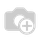• Pricing

# Odoo template for loop working unexpected.

Here is the template code where it doesn't work as expected. I expected [10, 10, 10] for foo array but insted it shows [0, 1, 2] when I display the output.

`< t t-set = "foo" t-value = "[]" />< t t-foreach = "[1,2,3]" t-as = "i" >  < t t-set = "foo" t-value = "foo + " />  < p > < t t-esc = "i" /> </ p > </ t >the below piece of code also throws an error of No append function.< t t-set = "foo" t-value = "[]" /> < t t-set = "foo" t-value = "foo.append(10)"/>`